Latest Banking jobs   »

# Quantitative Aptitude Quiz For Bank Foundation 2023 -20th May

Directions (1-5): In each of these questions, two equations (I) and (II) are given. You have to solve both the equations and give answer
(a) if x>y
(b) if x≥y
(c) if x = y or no relation can be established between x and y.
(d) if y>x
(e) if y≥x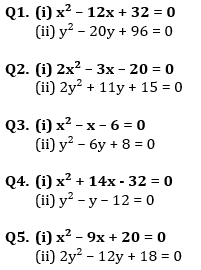Q6. The population of a town 3,11,250. The ratio of women and men is 43 : 40. If there are 60% literate among men, the total number of illiterate men in the town is :
(a) 80,000
(b) 60,000
(c) 90,000
(d) 70,000
(e) 68,000

Q7. The price of a refrigerator and a television set are in the ratio 5 : 3. If the refrigerator costs Rs. 5500 more than the television set, then the price of the refrigerator is :
(a) Rs. 27500
(b) Rs 8250
(c) Rs 13750
(d) Rs 16500
(e) Rs 15500

Q8. The marked price of a watch is Rs 1,600. The shopkeeper gives successive discount of 10% and x% to the customer. If the customer pays Rs 1,224 for the watch, the value of x is
(a) 5%
(b) 10%
(c) 15%
(d) 20%
(e) 18%

Q9. A box contains Rs 56 in the form of coins of one rupee, 50 paise and 25 paise. The number of 50 paise coins is double the number of 25 paise coins and four times the number of one-rupee coins. How many 50 paise coins are there in the box?
(a) 52
(b) 64
(c) 32
(d) 16
(e) 18

Q10. A skilled, a half skilled and an unskilled laborer work for 7, 8 and 10 days respectively and they together get Rs 396 for their work. If the ratio of their each day’s work is 1/3:1/4:1/6, then how much does the skilled labourer get (in rupees)?
(a) 164
(b) 162
(c) 145
(d) 154
(e) 126

Solutions: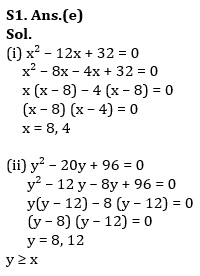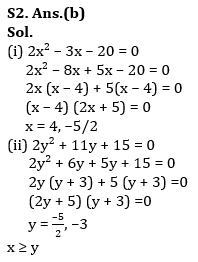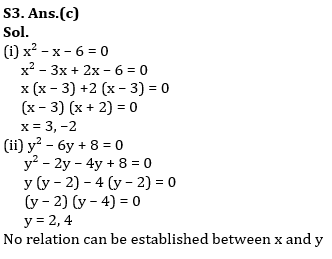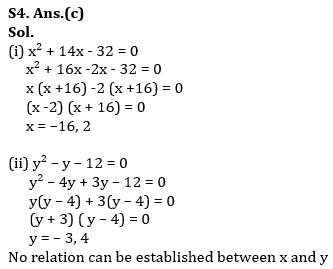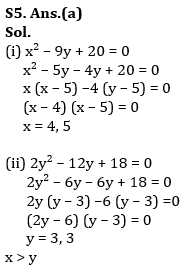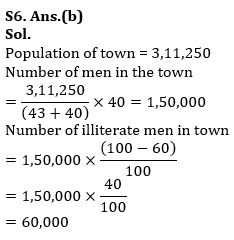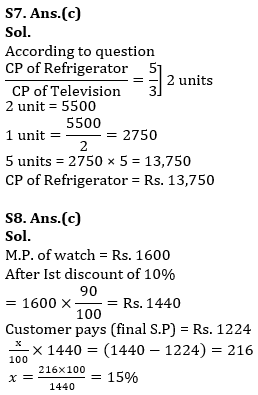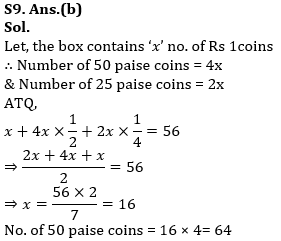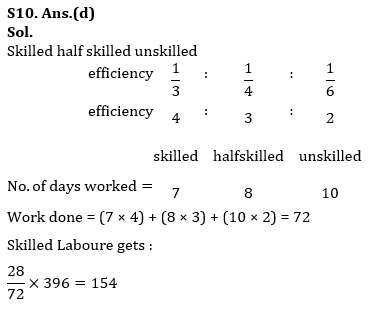## FAQs

### What is the selection process of the Bank Clerk?

The selection process of the Bank Clerk is Prelims & Mains.

#### Congratulations!Union Budget 2023-24: Free PDF